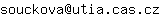Ústav teorie informace a automatizace

## Optimální PWM problém

Petr Kujan
Typ obhajoby:
Ph.D.
Datum konání:
23.10.2009
Místo:
ČVUT FEL v Praze, Katedra řídicí techniky, Karlovo nám. 13, 121 35 Praha 2, budova E v areálu Strojní fakulty, místnost 14
Mail:Stav:
obhájeno
The thesis deals with optimal pulse width modulation (PWM) problem which is an established method of generating PWM waveforms (or general multilevel waveforms) with low baseband distortion. We focused on optimal computation of switching times of a PWM waveform for generating general odd symmetric signals with applications in control. We introduce an exact and fast algorithm with the complexity of only O(n log^2 n) operations. This algorithm is based on converting appropriate trigonometric equations of each harmonics to a polynomial system of equations that is in turn transferred into a special system of power sums. The solution of this system is carried out by a modification of Newton’s identity, Pade approximation theory, formal orthogonal polynomials theory and properties of symmetric polynomials. Finally, the optimal switching sequence is obtained by computing the zeros of two formal orthogonal univariate polynomials. Application cover control of large electric drives, power electronics converters, active harmonic filters, optimal (micro) electro-mechanical systems or digital audio amplifier. Implementation of fast and efficient proposed algorithms on dedicated hardware, e.g. digital signal processor, opens a possibility of better on-the-fly construction and more accurate and faster solutions. It can result in increasing full or power efficiency, lower emissions, and better performance.
03.05.2018 - 08:01